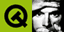DOC HOME SITE MAP MAN PAGES GNU INFO SEARCH PRINT BOOK
 Home | All Classes | Main Classes | Annotated | Grouped Classes | Functions# QDoubleValidator Class Reference

The QDoubleValidator class provides range checking of floating-point numbers. More...

#include <qvalidator.h>

Inherits QValidator.

## Properties

• double bottom - the validator's minimum acceptable value
• int decimals - the validator's maximum number of digits after the decimal point
• double top - the validator's maximum acceptable value

## Detailed Description

The QDoubleValidator class provides range checking of floating-point numbers.

QDoubleValidator provides an upper bound, a lower bound and a limit on the number of digits after the decimal point. It does not provide a fixup() function.

You can set the acceptable range in one call with setRange(), or with setBottom() and setTop(). Set the number of decimal places with setDecimals(). The validate() function returns the validation state.

## Member Function Documentation

### QDoubleValidator::QDoubleValidator ( QObject * parent, const char * name = 0 )

Constructs a validator object with parent parent, called name, which accepts any double.

### QDoubleValidator::QDoubleValidator ( double bottom, double top, int decimals, QObject * parent, const char * name = 0 )

Constructs a validator object with parent parent, called name. This validator will accept doubles from bottom to top inclusive, with up to decimals digits after the decimal point.

### QDoubleValidator::~QDoubleValidator ()

Destroys the validator, freeing any resources used.

### double QDoubleValidator::bottom () const

Returns the validator's minimum acceptable value. See the "bottom" property for details.

### int QDoubleValidator::decimals () const

Returns the validator's maximum number of digits after the decimal point. See the "decimals" property for details.

### void QDoubleValidator::setBottom ( double )

Sets the validator's minimum acceptable value. See the "bottom" property for details.

### void QDoubleValidator::setDecimals ( int )

Sets the validator's maximum number of digits after the decimal point. See the "decimals" property for details.

### void QDoubleValidator::setRange ( double minimum, double maximum, int decimals = 0 ) [virtual]

Sets the validator to accept doubles from minimum to maximum inclusive, with at most decimals digits after the decimal point.

### void QDoubleValidator::setTop ( double )

Sets the validator's maximum acceptable value. See the "top" property for details.

### double QDoubleValidator::top () const

Returns the validator's maximum acceptable value. See the "top" property for details.

### QValidator::StateQDoubleValidator::validate ( QString & input, int & ) const [virtual]

Returns Acceptable if the string input contains a double that is within the valid range and is in the correct format.

Returns Intermediate if input contains a double that is outside the range or is in the wrong format, e.g. with too many digits after the decimal point or is empty.

Returns Invalid if the input is not a double.

Note: If the valid range consists of just positive doubles (e.g. 0.0 - 100.0) and input is a negative double then Invalid is returned.

Reimplemented from QValidator.

## Property Documentation

### double bottom

This property holds the validator's minimum acceptable value.

Set this property's value with setBottom() and get this property's value with bottom().

### int decimals

This property holds the validator's maximum number of digits after the decimal point.

Set this property's value with setDecimals() and get this property's value with decimals().

### double top

This property holds the validator's maximum acceptable value.

Set this property's value with setTop() and get this property's value with top().